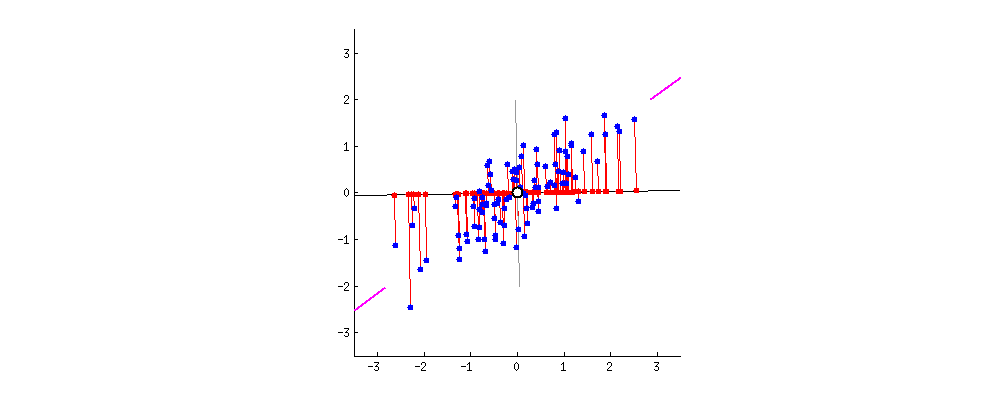# PCA的简单推导

PCA有两种通俗易懂的解释，1)是最大化投影后数据的方差(让数据更分散)2)是最小化投影造成的损失。这两个思路最后都能推导出同样的结果。Jj=1mi=1m(xTiuj)2=1m(xTuj)2=1m(xTuj)T(xTuj)=1muTjxxTuj

1mxxT$\frac1mxx^T$记作S$S$，假设我们要减去t个维度，则需要最小化
J=j=ntnuTjSujs.t.uTjuj=1

J~=j=ntnuTjSuj+λj(1uTjuj)

δJ~δuj=Sujλjuj

Suj=λjuj

J=j=ntnuTjSuj=j=ntnuTjλjuj=j=ntnλj

1. 对数据去中心化
2. 计算XXT$XX^T$，注:这里除或不除样本数量M$M$M1$M-1$其实对求出的特征向量没影响
3. XXT$XX^T$进行特征分解
4. 选取特征值最大的几个维度进行数据映射。（去掉较小的维度）

## 遗留问题

Σ=E[(xE[x])(xE[x])]

def pca_01(X):
covMat = np.cov(X,rowvar = 0)
eigVal,eigVec = sp.linalg.eig(covMat)
#do reduction with eigVal,eigVec


def pca_02(X):
mean_ = np.mean(X, axis=0)
X = X - mean_
covMat = np.cov(X,rowvar = 0)#实际上是否去中心化对求到的协方差矩阵并无影响,只是方便后面进行降维
eigVal,eigVec = sp.linalg.eig(covMat)
#do reduction with eigVal,eigVec


def pca_03(X):
mean_ = np.mean(X, axis=0)
X = X - mean_
M,N=X.shape
Sigma=np.dot(X.transpose(),X)/(M-1)
eigVal,eigVec = sp.linalg.eig(Sigma)
#do reduction with eigVal,eigVec


# 这跟SVD有啥关系？

X=UΣV,$X=U\Sigma V^{*},\,} \$

X=UΣV,$X=U\Sigma V^{*},\,} \$
1. V$V$的列（columns）组成一套对 X$X\,$的正交”输入”或”分析”的基向量。这些向量是 XTX${ X^{T}X}$的特征向量。
2. U$U$的列（columns）组成一套对 X$X\,$的正交”输出”的基向量。这些向量是XXT${ XX^{T}}$的特征向量。
3. Σ$Σ$对角线上的元素是奇异值，可视为是在输入与输出间进行的标量的”膨胀控制”。这些是XXT${ XX^{T}}$XTX${ X^{T}X}$的特征值的非零平方根，并与U和V的行向量相对应。

def pca_04(X):
mean_ = np.mean(X, axis=0)
X = X - mean_
M,N=X.shape
Sigma=np.dot(X.transpose(),X) #这里直接去掉/(M-1)方便和pca_05比较，对求得特征向量无影响
U,S,V = sp.linalg.svd(Sigma);
eigVal,eigVec = S,U
#do reduction with eigVal,eigVec


def pca_05(X):
mean_ = np.mean(X, axis=0)
X = X - mean_
U, S, V = sp.linalg.svd(X)
eigVal,eigVec = S,V
#do reduction with eigVal,eigVec


## 首先我们需要推导出结论2：

X=UΣVT

XTX=VΣUTUΣVT=VΣ2VT=VΣ2V1

Σ$\Sigma$是对角矩阵，U是标准正交基（酉矩阵），V是标准正交基（VVT=I;V=V1$VV^T=I;V=V^{-1}$

XTX=QΛQ1

V=QΛ=Σ2

PCA_04:
eigVal：[ 21.60311815 8.77188185]
eigVec： [[-0.88734696 -0.46110235]
[-0.46110235 0.88734696]]

PCA_05:
eigVal：[ 4.64791546 2.96173629]
eigVec： [[ 0.88734696 0.46110235]
[-0.46110235 0.88734696]]
#注意PCA_05结果中特征向量维度的符号，和上面不太一样，但这不影响降维的功能，每一列是一组基

## 对于结论一：

XTX=U2Σ2VT2

1. U$U$的列（columns）组成一套对 X$X\,$的正交”输出”的基向量。这些向量是XXT${ XX^{T}}$的特征向量。

XTXXTX=U2Σ2VT2(U2Σ2VT2)T=U2Σ22UT2

XTX=Q2Λ2Q12XTXXTX=Q2Λ22Q12

U2=Q2Σ2=Λ2

# PCA和SVD的应用

PCA是不必多说，一提到降维方法首先想到的就是PCA，关于降维方法后面可能还会找时间整理一些有意思的算法，我们可以看到对这些算法都有很intuitive的解释，搞懂是如何从intuition到公式再到计算步骤，是一个非常有意思的过程。如果只是停留在了解算法思想和流程，然后拿着库用一用，会丢掉很多有意思的东西。

SVD其实是众多矩阵分解的一种，除了在PCA上使用，也有用于推荐，在推荐领域的svd算法形式上并不能和标准的奇异值分解对应上，但其思路是相通的，具体可以参考协同过滤算法实现。同时SVD也可以很方便地算出矩阵的伪逆，这在最小二乘中有应用：

X1=VΣ1UT

# 总结

PCA有很好的直觉解释，一些可视化也很直观，所以往往忽视了其中的一些细节，深入地了解下来发现了很多有意思的东西，很有收获。笔者水平有限，如果文中有什么错误，还请告知，不甚感谢。